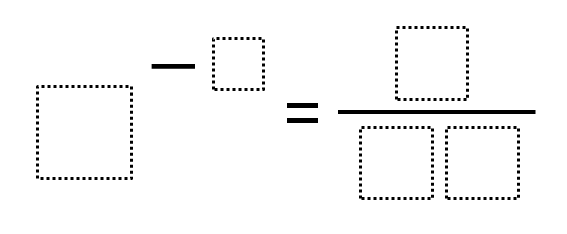Home > Grade 8 > Expressions & Equations > Negative Exponents – Closest to Zero

# Negative Exponents – Closest to Zero

Directions: Use the digits 0 to 9, at most one time each, to fill in the boxes to to make a result that is as close to zero as possible.### Hint

What happens when you have a negative exponent?
Where does the 1 go?

So far, there are two answers that have a result of 1/64.

8 ^ (-2) = 1 / 64
4 ^ (-3) = 1 / 64

Source: Daniel Luevanos

## Square Root Expression

Directions: Use the digits 1 to 9, at most one time each, to fill in …

1.How can you use the 4 in two places in the equation? If that’s allowed, then 2 ^ -6 = 1/64 should also be allowed.

2.I see you don’t monetize your website, don’t waste your traffic,
you can earn extra cash every month because you’ve got high quality content.

If you want to know how to make extra bucks, search for: Mrdalekjd methods for \$\$\$

3.So, it seems 7^-2 = 1/49 and 8^-2 = 1/64 are the only solutions which use unique digits, with the latter being closer to zero.